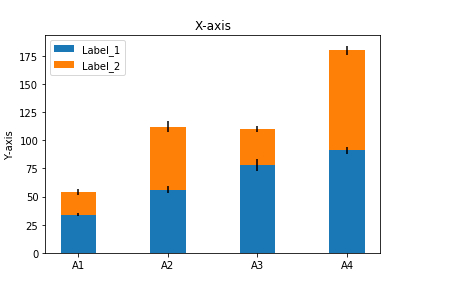# How to display stacked bar chart using matplotlib in Python?

Matplotlib is a popular Python package that is used for data visualization. Visualizing data is a key step since it helps understand what is going on in the data without actually looking at the numbers and performing complicated computations. It helps in communicating the quantitative insights to the audience effectively.

Matplotlib is used to create 2 dimensional plots with the data. It comes with an object oriented API that helps in embedding the plots in Python applications. Matplotlib can be used with IPython shells, Jupyter notebook, Spyder IDE and so on.

It is written in Python. It is created using Numpy, which is the Numerical Python package in Python.

Python can be installed on Windows using the below command −

pip install matplotlib

The dependencies of Matplotlib are −

Python ( greater than or equal to version 3.4)
NumPy
Setuptools
Pyparsing
Libpng
Pytz
Free type
Six
Cycler
Dateutil

Stacked bar plots show the data points of two values in a single rectangular box.

Let us understand how Matplotlib can be used to create a stacked plot −

## Example

import matplotlib.pyplot as plt
labels = ['A1', 'A2', 'A3', 'A4']
val_1 = [34, 56, 78, 91]
val_2 = [20, 56, 32, 89]
val_3 = [1, 3, 5, 3]
val_4 = [3, 5, 3, 4]
width = 0.40

fig, ax = plt.subplots()

ax.bar(labels, val_1, width, yerr=val_3, label='Label_1')
ax.bar(labels, val_2, width, yerr=val_4, bottom=val_1,
label='Label_2')

ax.set_ylabel('Y−axis')
ax.set_title('X−axis')
ax.legend()
plt.show()

## Output## Explanation

• The required packages are imported and its alias is defined for ease of use.

• The labels for the stacked chart and values for the labels/bars are defined.

• An empty figure is created using the ‘figure’ function.

• The ‘subplot’ function is used to create an area to plot the graph.

• The data is plotted using the ‘plot’ function.

• The set_xlabel, set_ylabel and set_title functions are used to provide labels for ‘X’ axis, ‘Y’ axis and title.

• The plot is defined as a bar chart by specifying ‘bar’.

• It is shown on the console using the ‘show’ function.